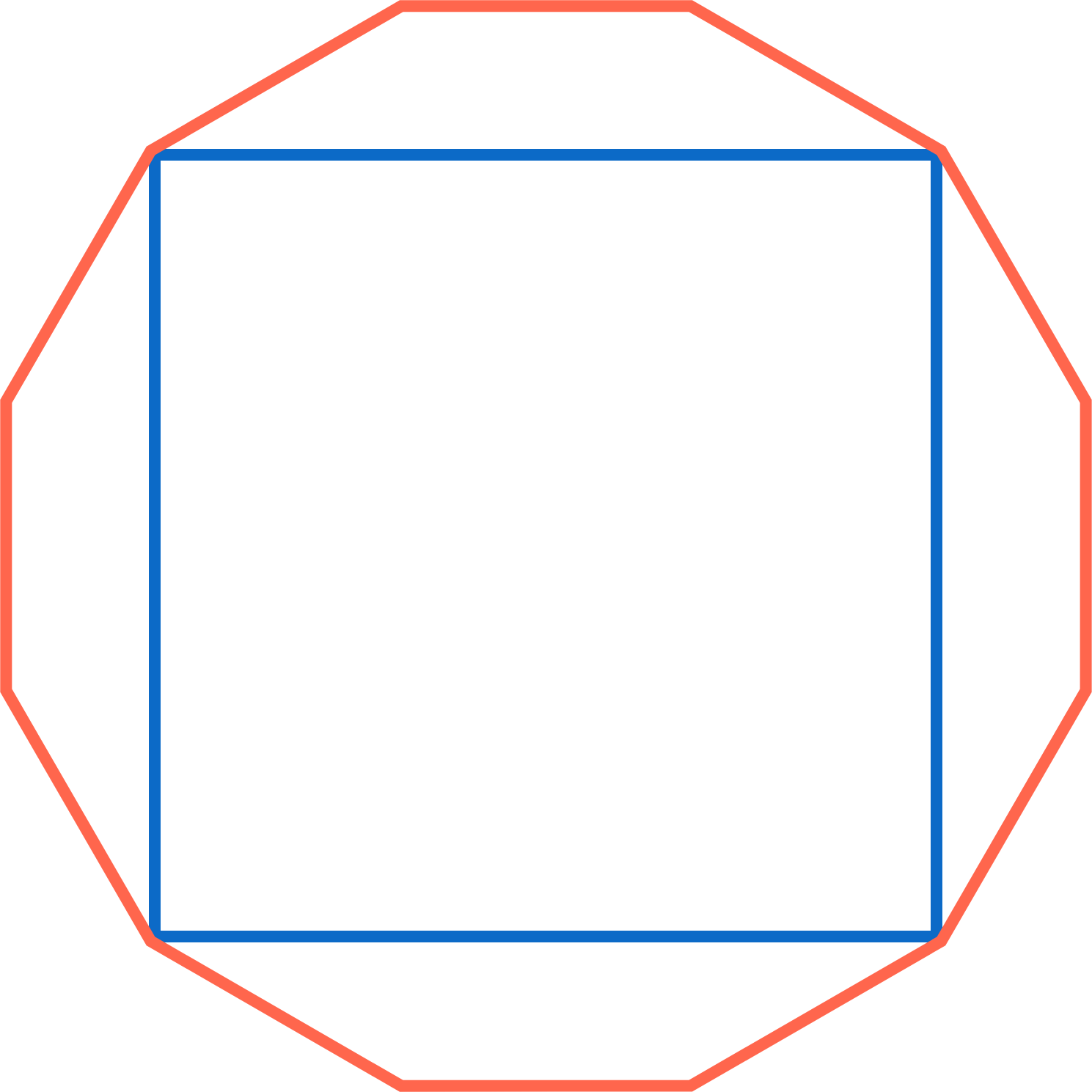A Square In A Dodecagon

Geometry Level 2A square is inscribed in a regular dodecagon--a regular polygon with 12 sides--so that they share $4$ vertices, as shown.

If the area of the dodecagon is $12$, what is the area of the square?

×